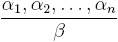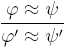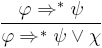Research activities

# Deduction rules

The deduction rule in the logical calculus of association rules is a relation of the formwhere α1, α2, , αn, β are association rules. This deduction rule is correct if it holds for each data matrix M: If α1, α2, , αn are true in M, then also β is true in M.

We are interested in correct deduction rules of the formwhere φ ψ and φ' ψ' are association rules.

Such deduction rules can be used namely in the following ways:

• To reduce the output of a data mining procedure: If the association rule φ ψ is a part of a data mining procedure output (i.e. it is true in an analysed data matrix) and ifis the correct deduction rule then it is not necessary to put the association rule φ' ψ' into the output. The used deduction rule must be transparent enough from the point of view of the user of the data mining procedure. An example of a simple deduction rule is a dereduction deduction rulethat is correct for each implicational quantifier * [Ha 78].

• To decrease the number of actually tested association rules: If the association rule φ ψ is true in the analysed data matrix and ifis the correct deduction rule, then it is not necessary to test φ' ψ'.

Thus it is reasonable to ask when the deduction rule of the formis correct. It can shown that there are several propositional formulas Φ, Ψ derived from φ, ψ, φ', ψ' such that this deduction rule is correct if and only if Φ and Ψ are tautologie of the propositional calculu [Ra 98A], [Ra 98C]. The propositiobnal formulas Φ, Ψ depends on the class of 4ft-quantifiers the quantifier belongs to.

Some of these deduction rules are applied in the procedure 4ft-Miner.